# Chapter 3 Univariate Graphs

Univariate graphs plot the distribution of data from a single variable. The variable can be categorical (e.g., race, sex) or quantitative (e.g., age, weight).

## 3.1 Categorical

The distribution of a single categorical variable is typically plotted with a bar chart, a pie chart, or (less commonly) a tree map.

### 3.1.1 Bar chart

The Marriage dataset contains the marriage records of 98 individuals in Mobile County, Alabama. Below, a bar chart is used to display the distribution of wedding participants by race.

library(ggplot2)
data(Marriage, package = "mosaicData")

# plot the distribution of race
ggplot(Marriage, aes(x = race)) +
geom_bar()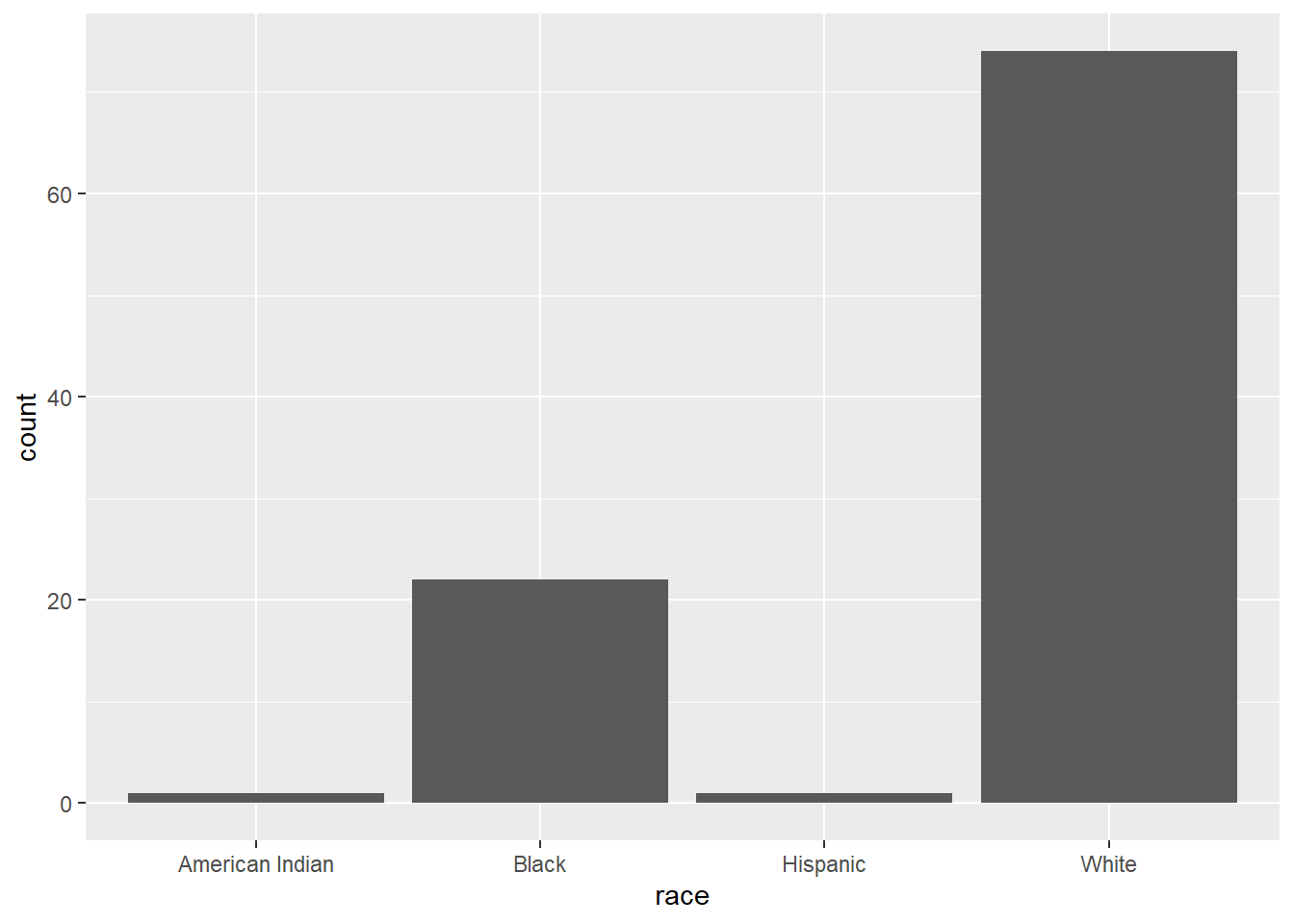Figure 3.1: Simple barchart

The majority of participants are white, followed by black, with very few Hispanics or American Indians.

You can modify the bar fill and border colors, plot labels, and title by adding options to the geom_bar function.

# plot the distribution of race with modified colors and labels
ggplot(Marriage, aes(x = race)) +
geom_bar(fill = "cornflowerblue",
color="black") +
labs(x = "Race",
y = "Frequency",
title = "Participants by race")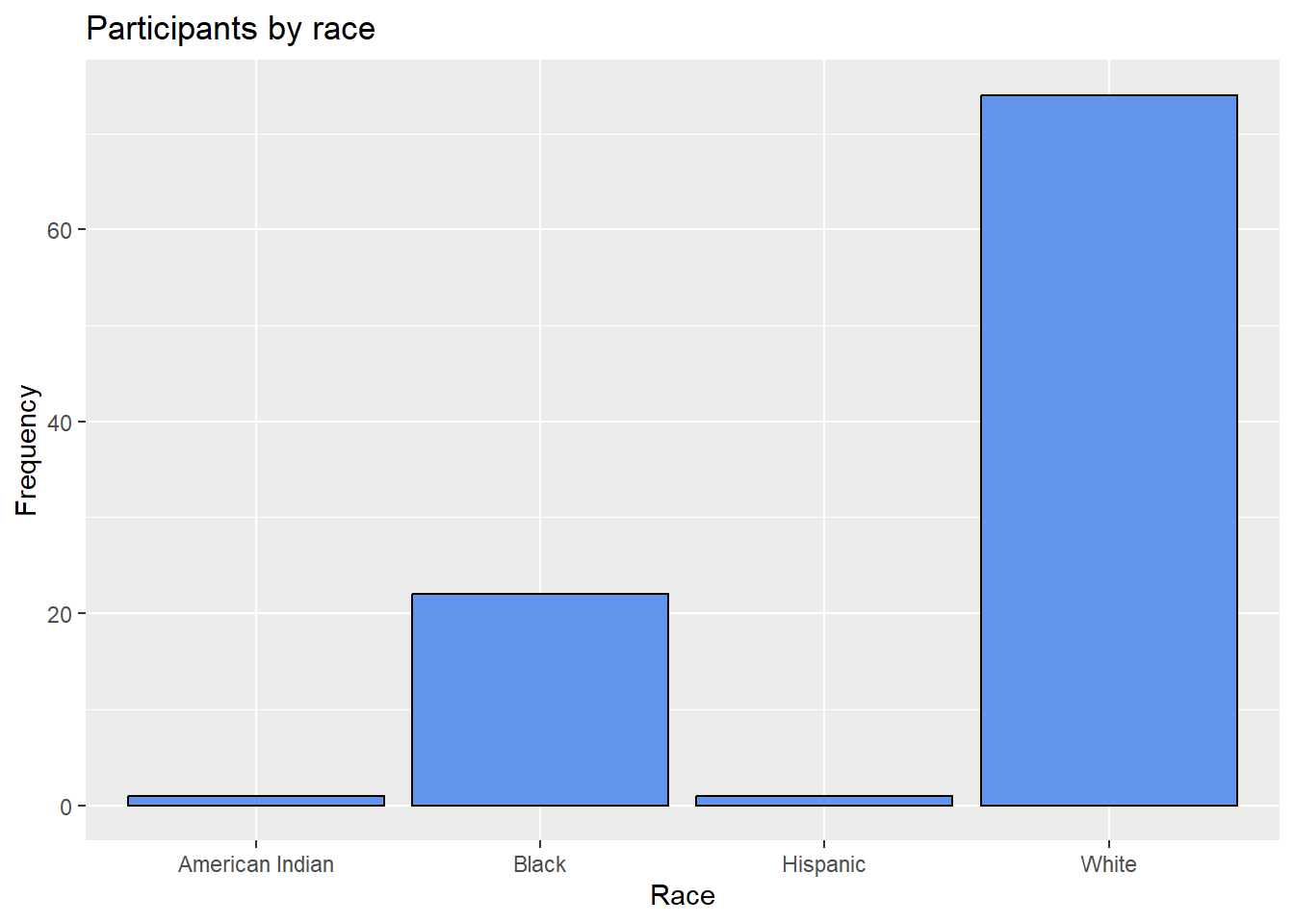Figure 3.2: Barchart with modified colors, labels, and title

#### 3.1.1.1 Percents

Bars can represent percents rather than counts. For bar charts, the code aes(x=race) is actually a shortcut for aes(x = race, y = ..count..), where ..count.. is a special variable representing the frequency within each category. You can use this to calculate percentages, by specifying the y variable explicitly.

# plot the distribution as percentages
ggplot(Marriage,
aes(x = race,
y = ..count.. / sum(..count..))) +
geom_bar() +
labs(x = "Race",
y = "Percent",
title  = "Participants by race") +
scale_y_continuous(labels = scales::percent)Figure 3.3: Barchart with percentages

In the code above, the scales package is used to add % symbols to the y-axis labels.

#### 3.1.1.2 Sorting categories

It is often helpful to sort the bars by frequency. In the code below, the frequencies are calculated explicitly. Then the reorder function is used to sort the categories by the frequency. The option stat="identity" tells the plotting function not to calculate counts, because they are supplied directly.

# calculate number of participants in
# each race category
library(dplyr)
plotdata <- Marriage %>%
count(race)

The resulting dataset is give below.

Table 3.1: plotdata
race n
American Indian 1
Black 22
Hispanic 1
White 74

This new dataset is then used to create the graph.

# plot the bars in ascending order
ggplot(plotdata,
aes(x = reorder(race, n),
y = n)) +
geom_bar(stat = "identity") +
labs(x = "Race",
y = "Frequency",
title  = "Participants by race")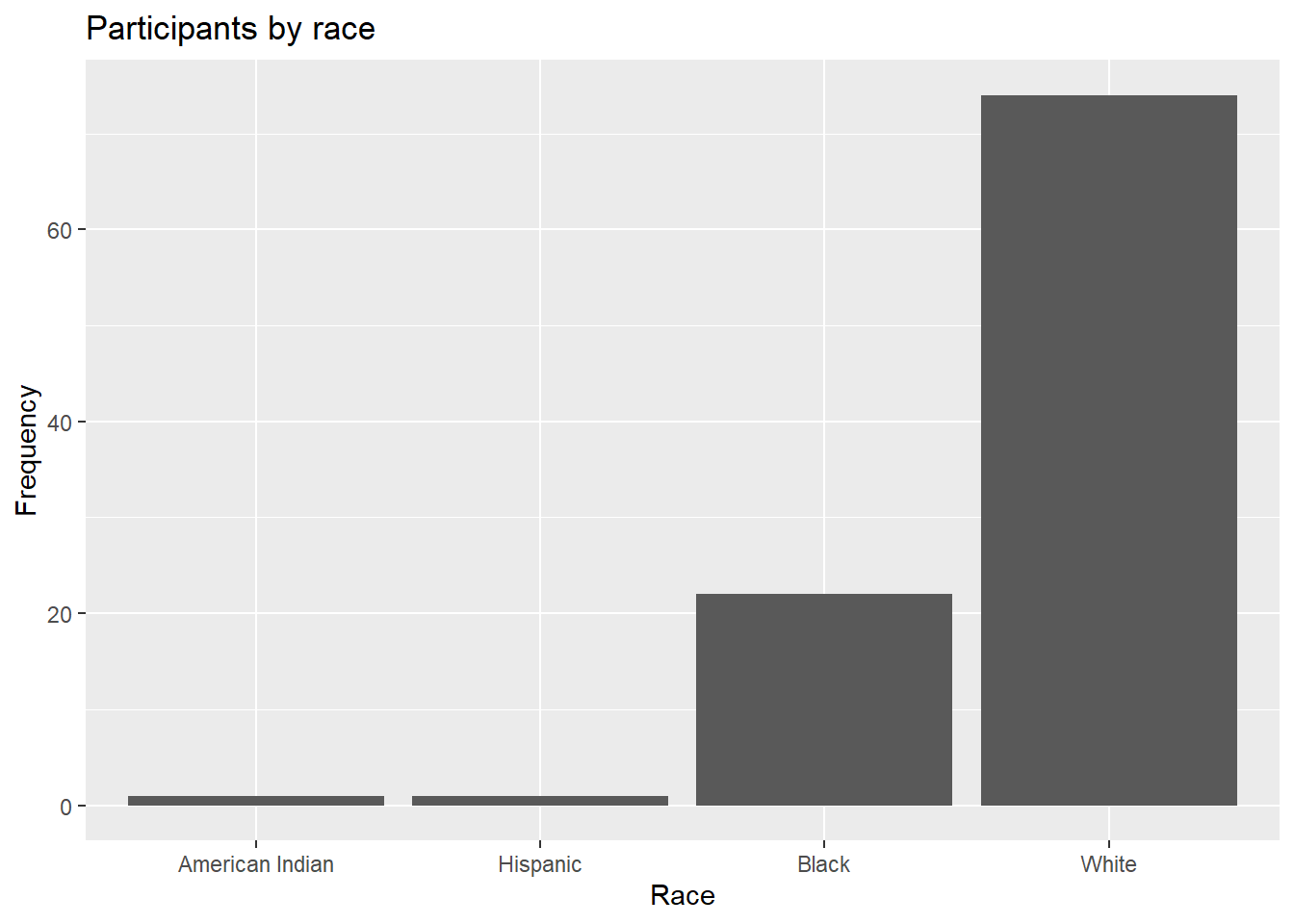Figure 3.4: Sorted bar chart

The graph bars are sorted in ascending order. Use reorder(race, -n) to sort in descending order.

#### 3.1.1.3 Labeling bars

Finally, you may want to label each bar with its numerical value.

# plot the bars with numeric labels
ggplot(plotdata,
aes(x = race,
y = n)) +
geom_bar(stat = "identity") +
geom_text(aes(label = n),
vjust=-0.5) +
labs(x = "Race",
y = "Frequency",
title  = "Participants by race")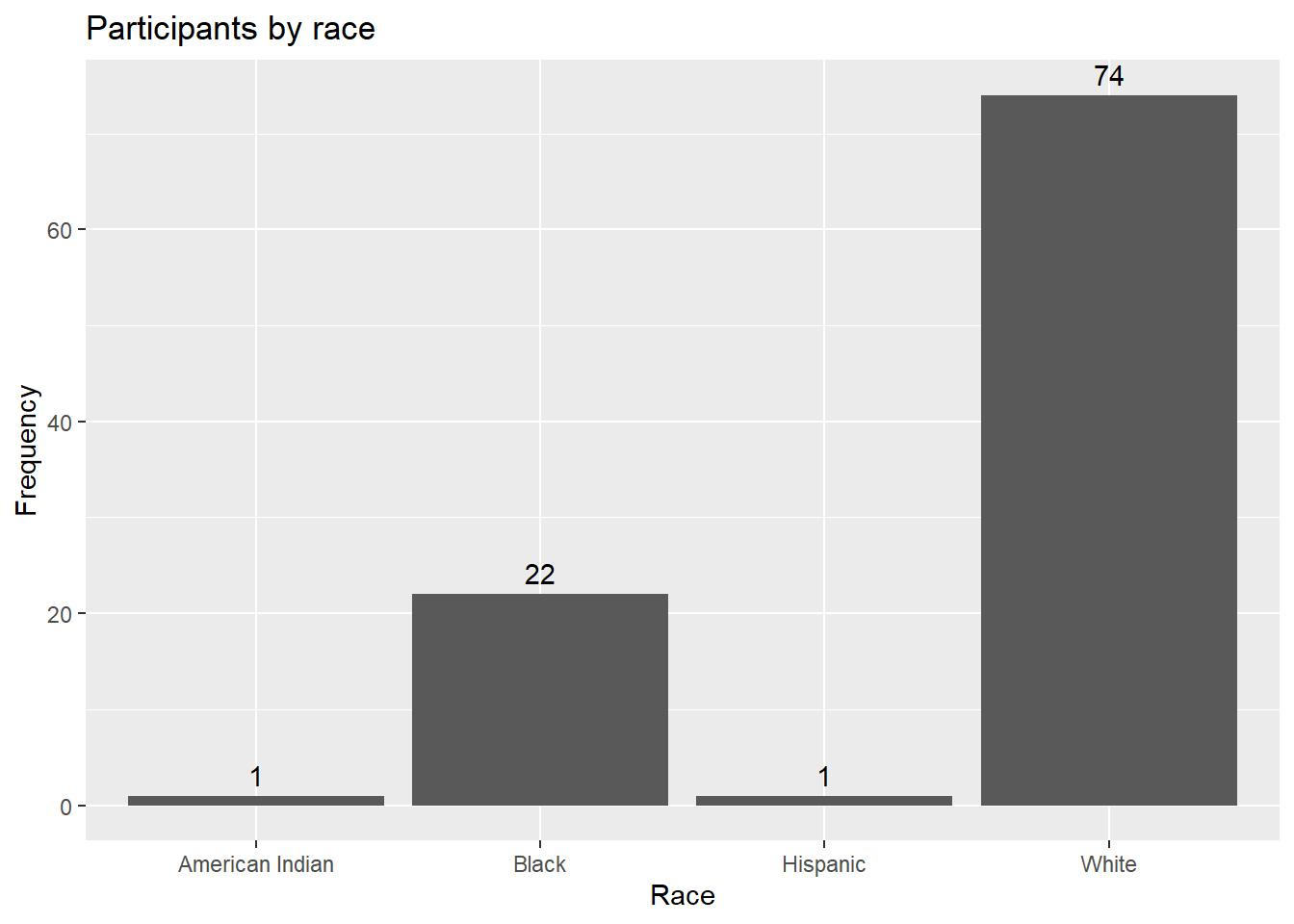Figure 3.5: Bar chart with numeric labels

Here geom_text adds the labels, and vjust controls vertical justification. See Annotations for more details.

Putting these ideas together, you can create a graph like the one below. The minus sign in reorder(race, -pct) is used to order the bars in descending order.

library(dplyr)
library(scales)
plotdata <- Marriage %>%
count(race) %>%
mutate(pct = n / sum(n),
pctlabel = paste0(round(pct*100), "%"))

# plot the bars as percentages,
# in decending order with bar labels
ggplot(plotdata,
aes(x = reorder(race, -pct),
y = pct)) +
geom_bar(stat = "identity",
fill = "indianred3",
color = "black") +
geom_text(aes(label = pctlabel),
vjust = -0.25) +
scale_y_continuous(labels = percent) +
labs(x = "Race",
y = "Percent",
title  = "Participants by race")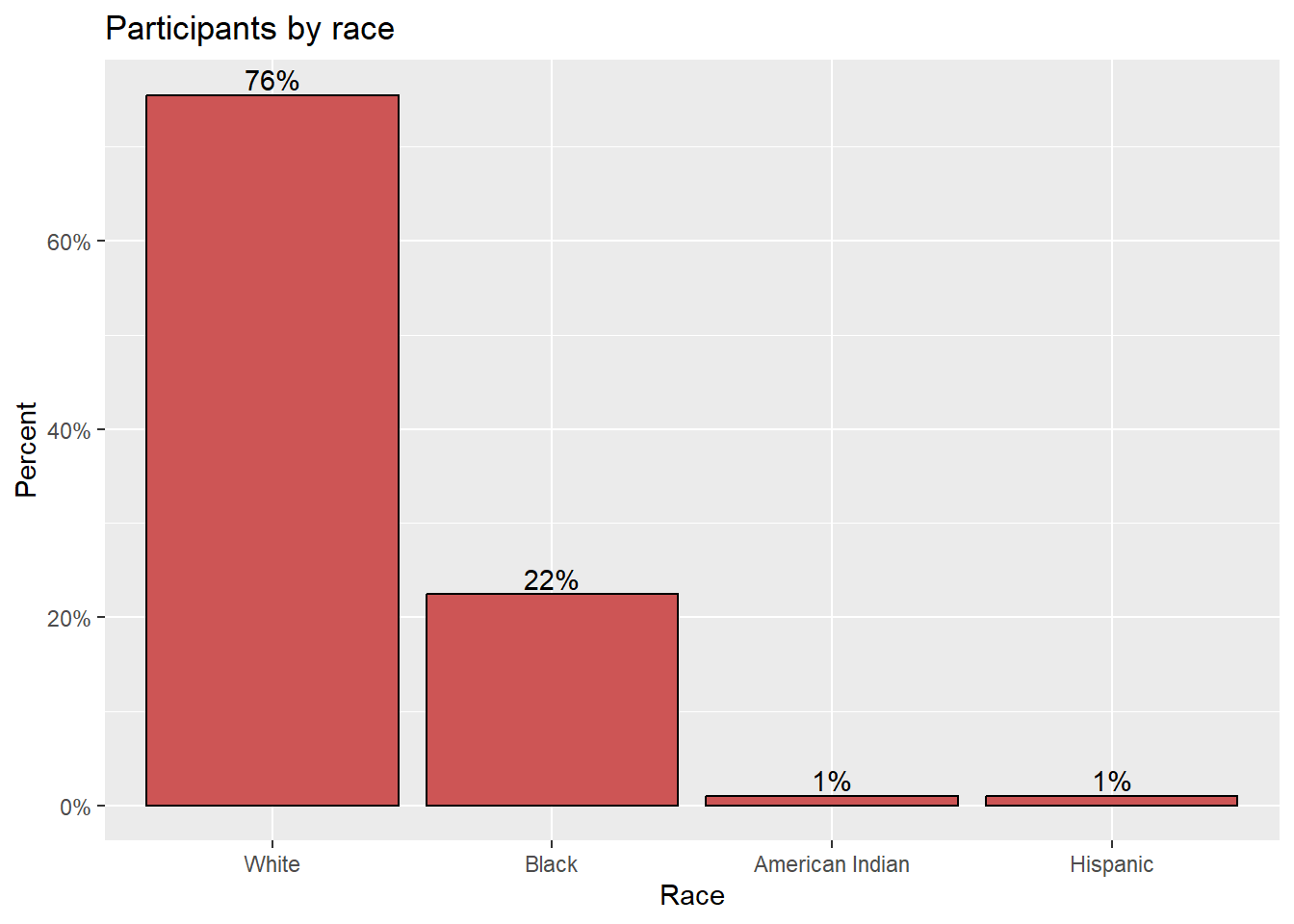Figure 3.6: Sorted bar chart with percent labels

#### 3.1.1.4 Overlapping labels

Category labels may overlap if (1) there are many categories or (2) the labels are long. Consider the distribution of marriage officials.

# basic bar chart with overlapping labels
ggplot(Marriage, aes(x = officialTitle)) +
geom_bar() +
labs(x = "Officiate",
y = "Frequency",
title = "Marriages by officiate")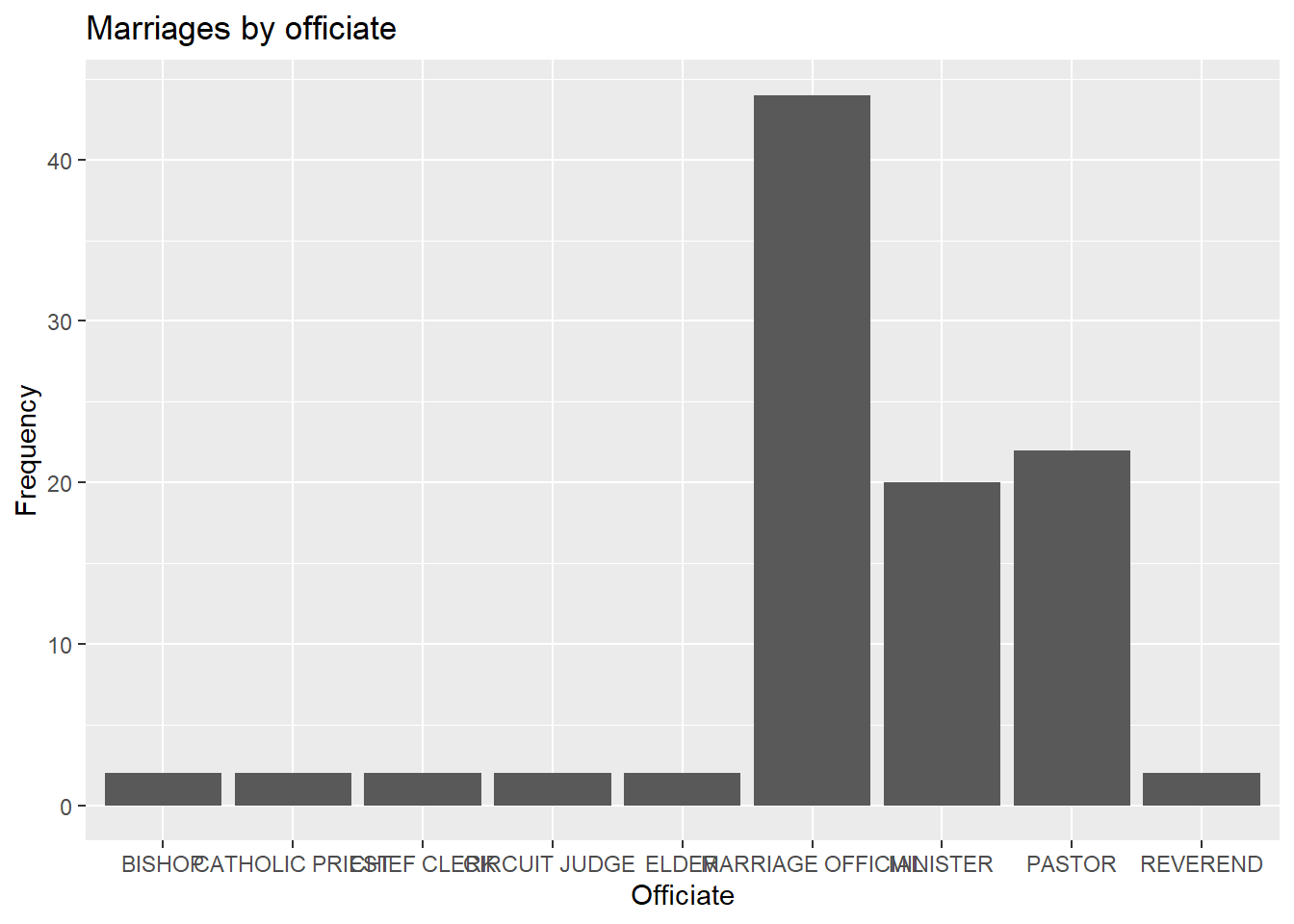Figure 3.7: Barchart with problematic labels

In this case, you can flip the x and y axes.

# horizontal bar chart
ggplot(Marriage, aes(x = officialTitle)) +
geom_bar() +
labs(x = "",
y = "Frequency",
title = "Marriages by officiate") +
coord_flip()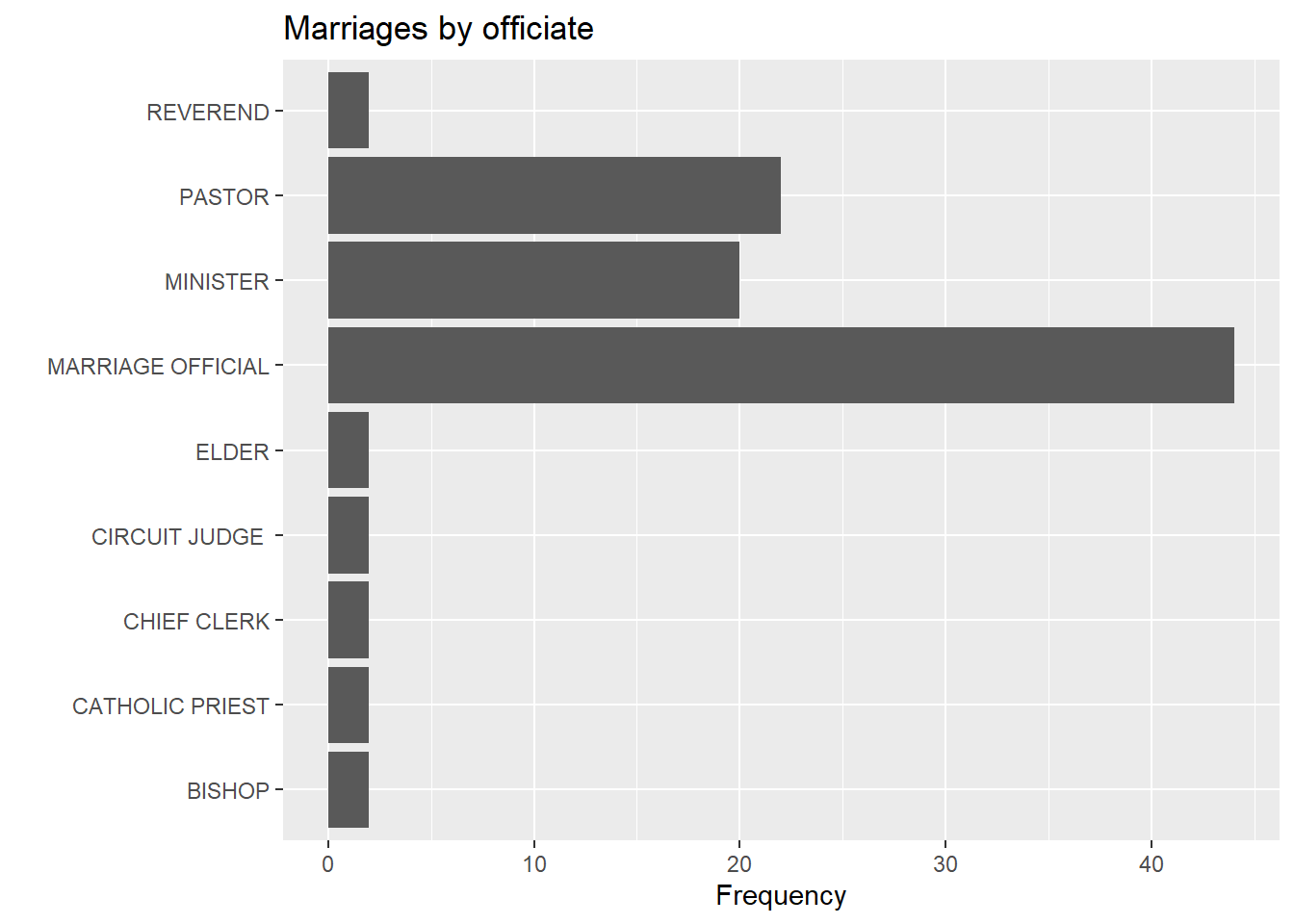Figure 3.8: Horizontal barchart

Alternatively, you can rotate the axis labels.

# bar chart with rotated labels
ggplot(Marriage, aes(x = officialTitle)) +
geom_bar() +
labs(x = "",
y = "Frequency",
title = "Marriages by officiate") +
theme(axis.text.x = element_text(angle = 45,
hjust = 1))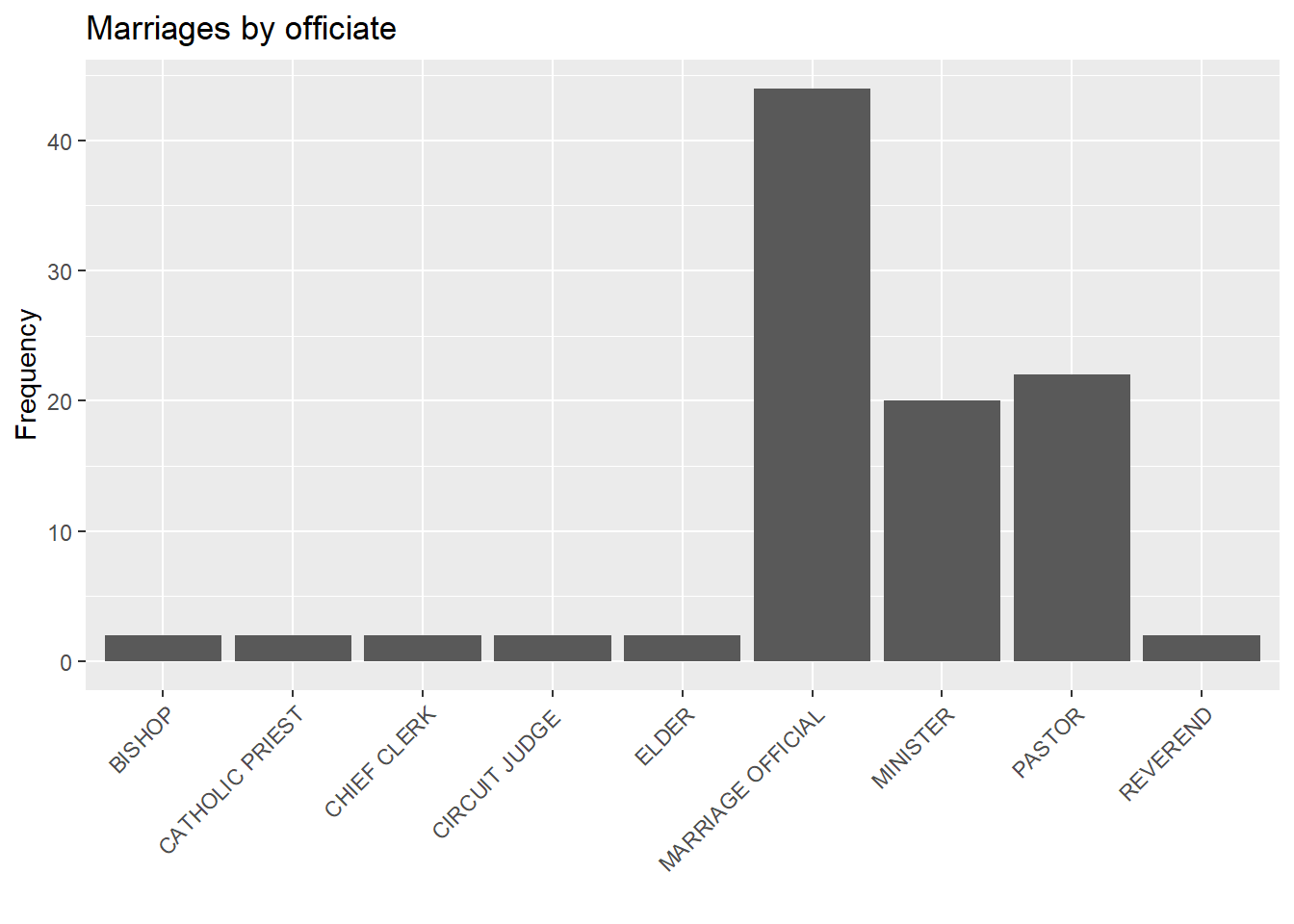Figure 3.9: Barchart with rotated labels

Finally, you can try staggering the labels. The trick is to add a newline \n to every other label.

# bar chart with staggered labels
lbls <- paste0(c("", "\n"),
levels(Marriage$officialTitle)) ggplot(Marriage, aes(x=factor(officialTitle, labels = lbls))) + geom_bar() + labs(x = "", y = "Frequency", title = "Marriages by officiate")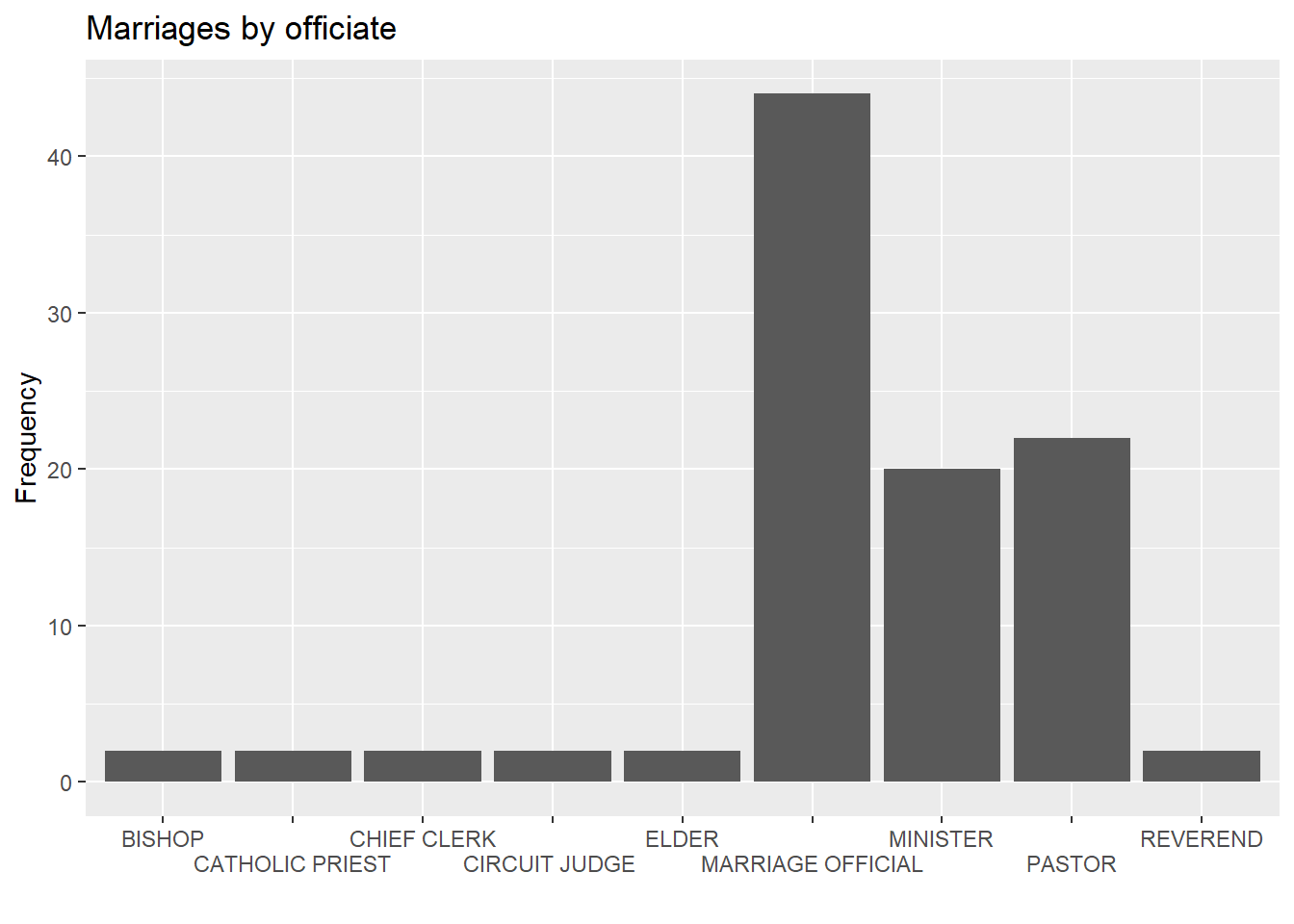Figure 3.10: Barchart with staggered labels ### 3.1.2 Pie chart Pie charts are controversial in statistics. If your goal is to compare the frequency of categories, you are better off with bar charts (humans are better at judging the length of bars than the volume of pie slices). If your goal is compare each category with the the whole (e.g., what portion of participants are Hispanic compared to all participants), and the number of categories is small, then pie charts may work for you. It takes a bit more code to make an attractive pie chart in R. # create a basic ggplot2 pie chart plotdata <- Marriage %>% count(race) %>% arrange(desc(race)) %>% mutate(prop = round(n * 100 / sum(n), 1), lab.ypos = cumsum(prop) - 0.5 *prop) ggplot(plotdata, aes(x = "", y = prop, fill = race)) + geom_bar(width = 1, stat = "identity", color = "black") + coord_polar("y", start = 0, direction = -1) + theme_void()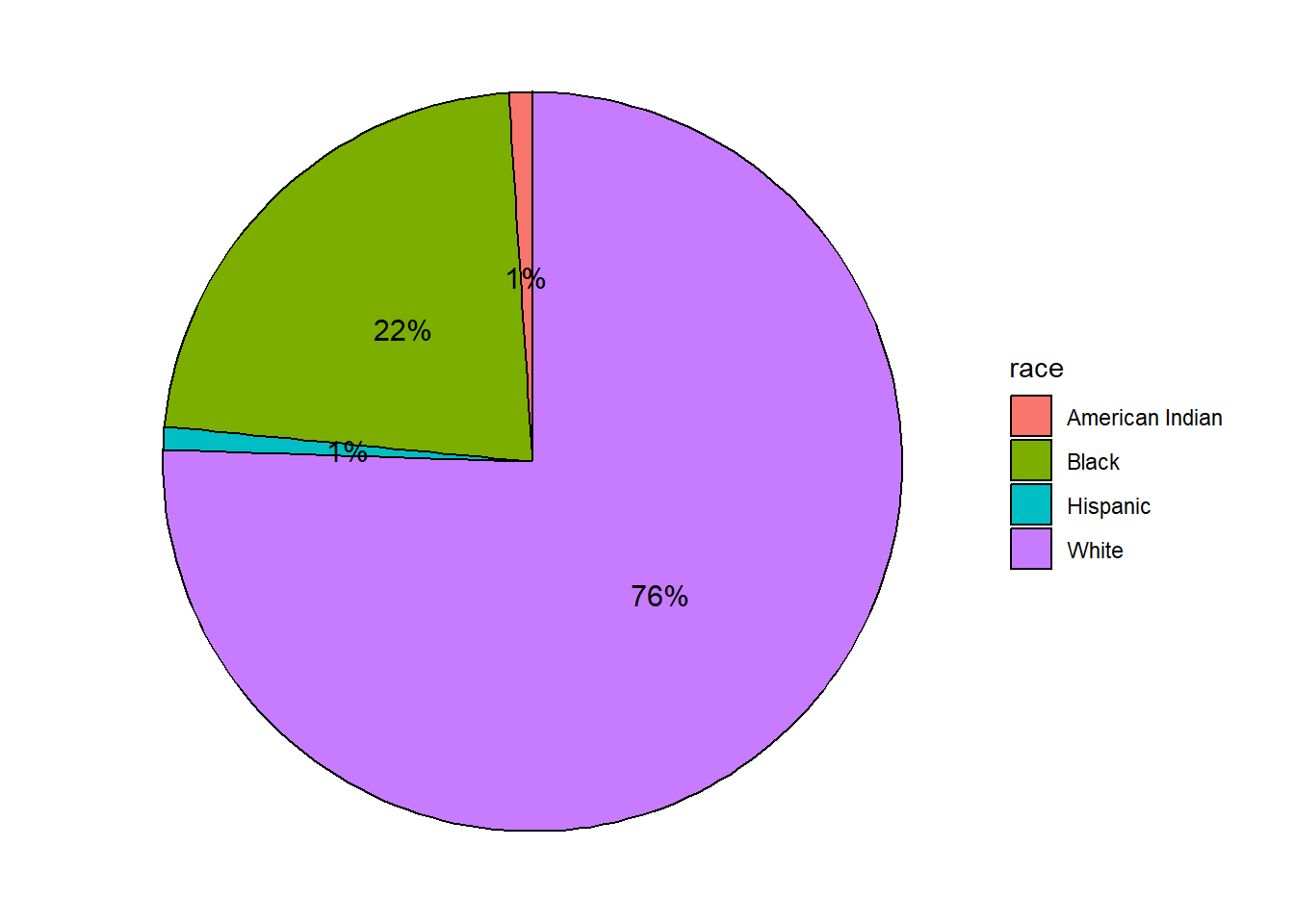Figure 3.11: Basic pie chart Now let’s get fancy and add labels, while removing the legend. # create a pie chart with slice labels plotdata <- Marriage %>% count(race) %>% arrange(desc(race)) %>% mutate(prop = round(n*100/sum(n), 1), lab.ypos = cumsum(prop) - 0.5*prop) plotdata$label <- paste0(plotdata$race, "\n", round(plotdata$prop), "%")

ggplot(plotdata,
aes(x = "",
y = prop,
fill = race)) +
geom_bar(width = 1,
stat = "identity",
color = "black") +
geom_text(aes(y = lab.ypos, label = label),
color = "black") +
coord_polar("y",
start = 0,
direction = -1) +
theme_void() +
theme(legend.position = "FALSE") +
labs(title = "Participants by race")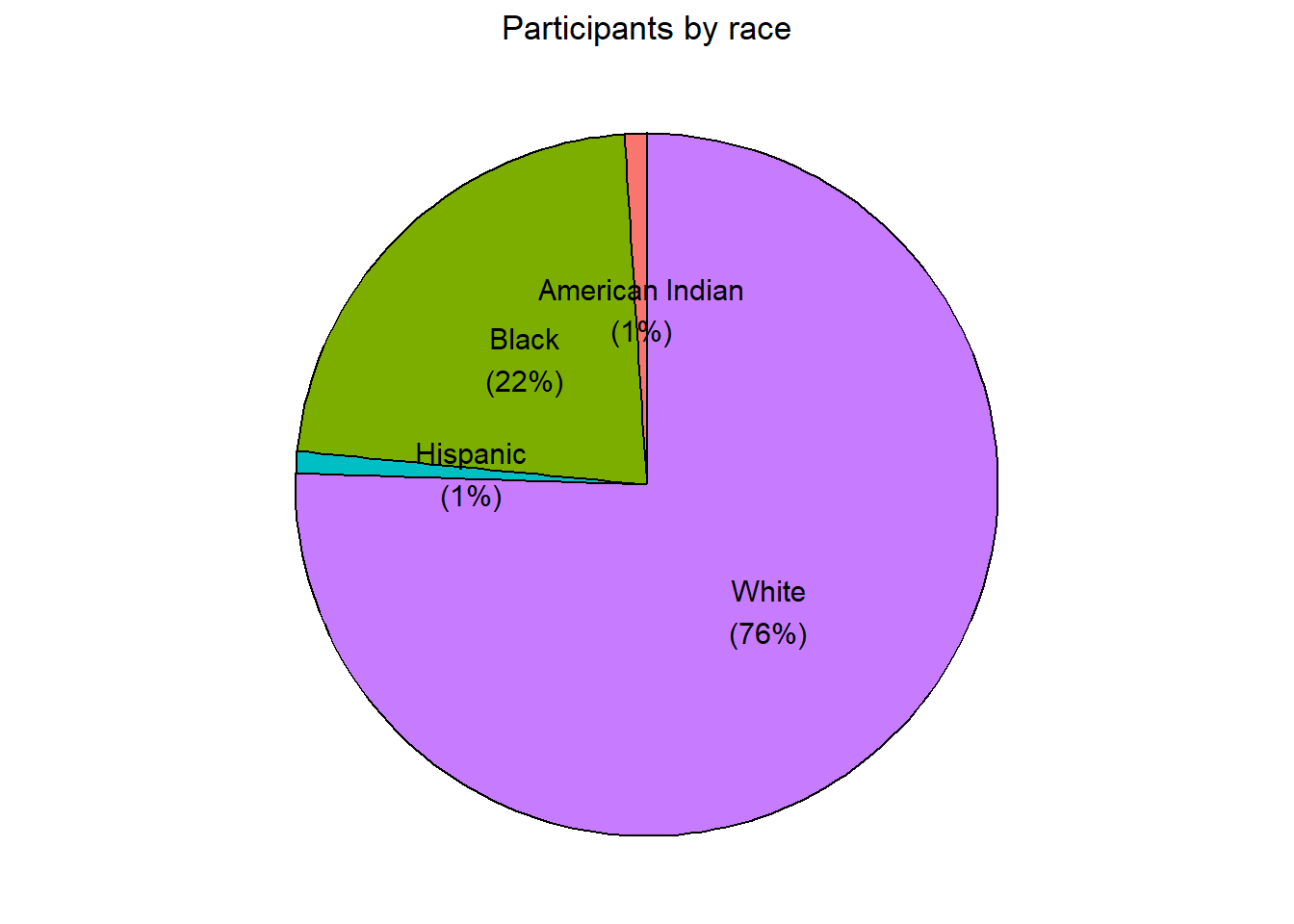Figure 3.12: Pie chart with percent labels

The pie chart makes it easy to compare each slice with the whole. For example, Back is seen to roughly a quarter of the total participants.

### 3.1.3 Tree map

An alternative to a pie chart is a tree map. Unlike pie charts, it can handle categorical variables that have many levels.

library(treemapify)

# create a treemap of marriage officials
plotdata <- Marriage %>%
count(officialTitle)

ggplot(plotdata,
aes(fill = officialTitle,
area = n)) +
geom_treemap() +
labs(title = "Marriages by officiate")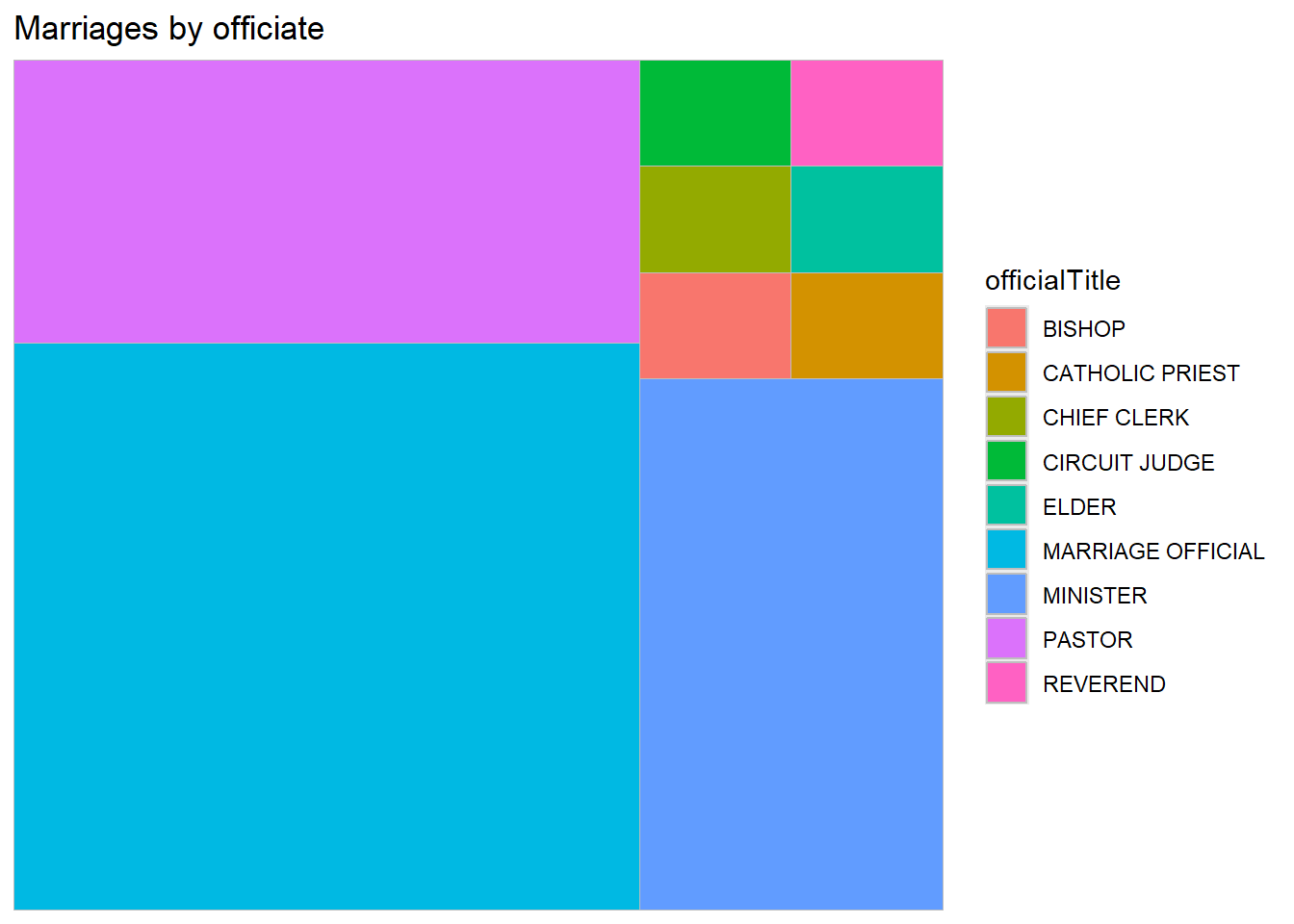Figure 3.13: Basic treemap

Here is a more useful version with labels.

# create a treemap with tile labels
ggplot(plotdata,
aes(fill = officialTitle,
area = n,
label = officialTitle)) +
geom_treemap() +
geom_treemap_text(colour = "white",
place = "centre") +
labs(title = "Marriages by officiate") +
theme(legend.position = "none")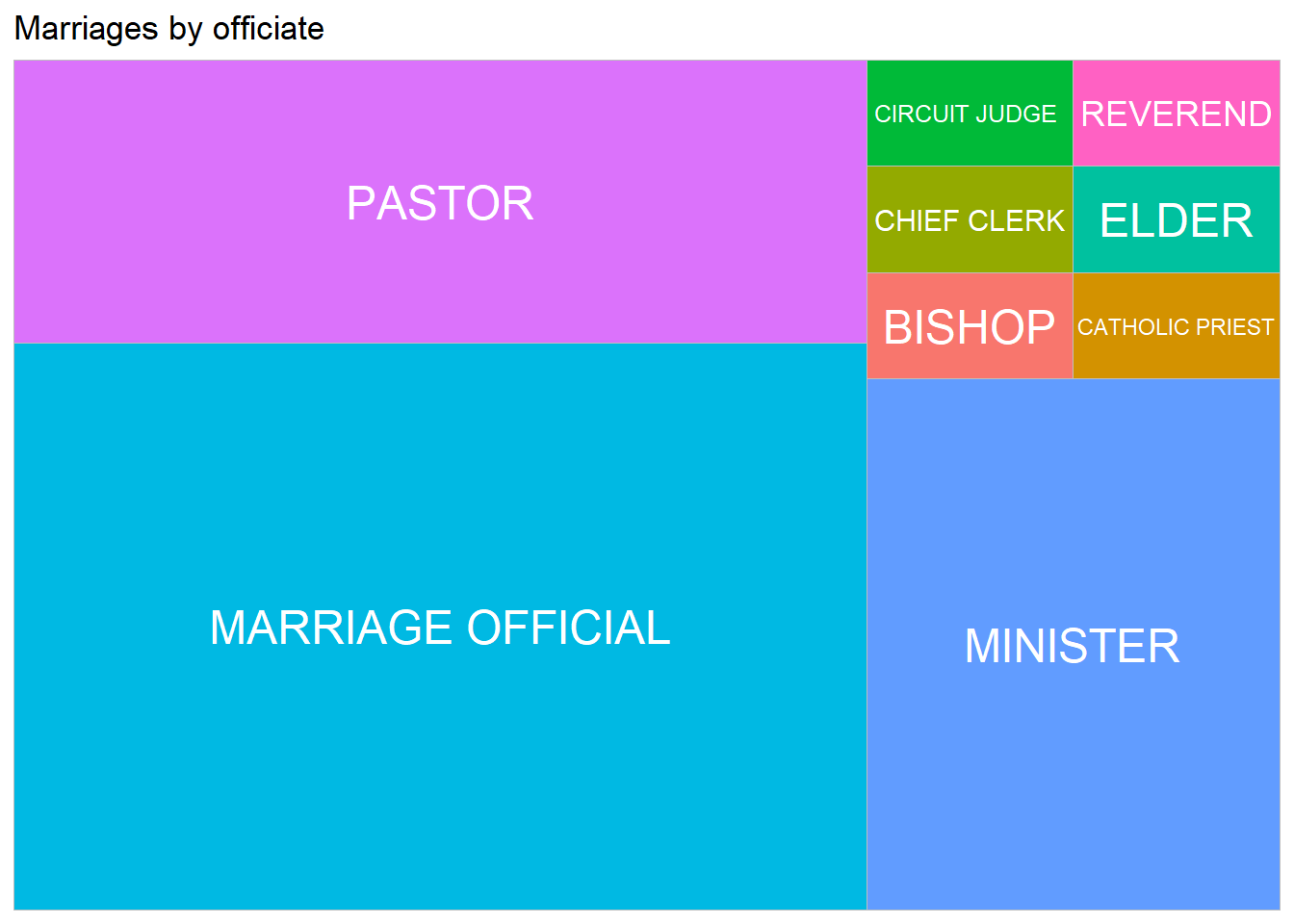Figure 3.14: Treemap with labels

## 3.2 Quantitative

The distribution of a single quantitative variable is typically plotted with a histogram, kernel density plot, or dot plot.

### 3.2.1 Histogram

Using the Marriage dataset, let’s plot the ages of the wedding participants.

library(ggplot2)

# plot the age distribution using a histogram
ggplot(Marriage, aes(x = age)) +
geom_histogram() +
labs(title = "Participants by age",
x = "Age")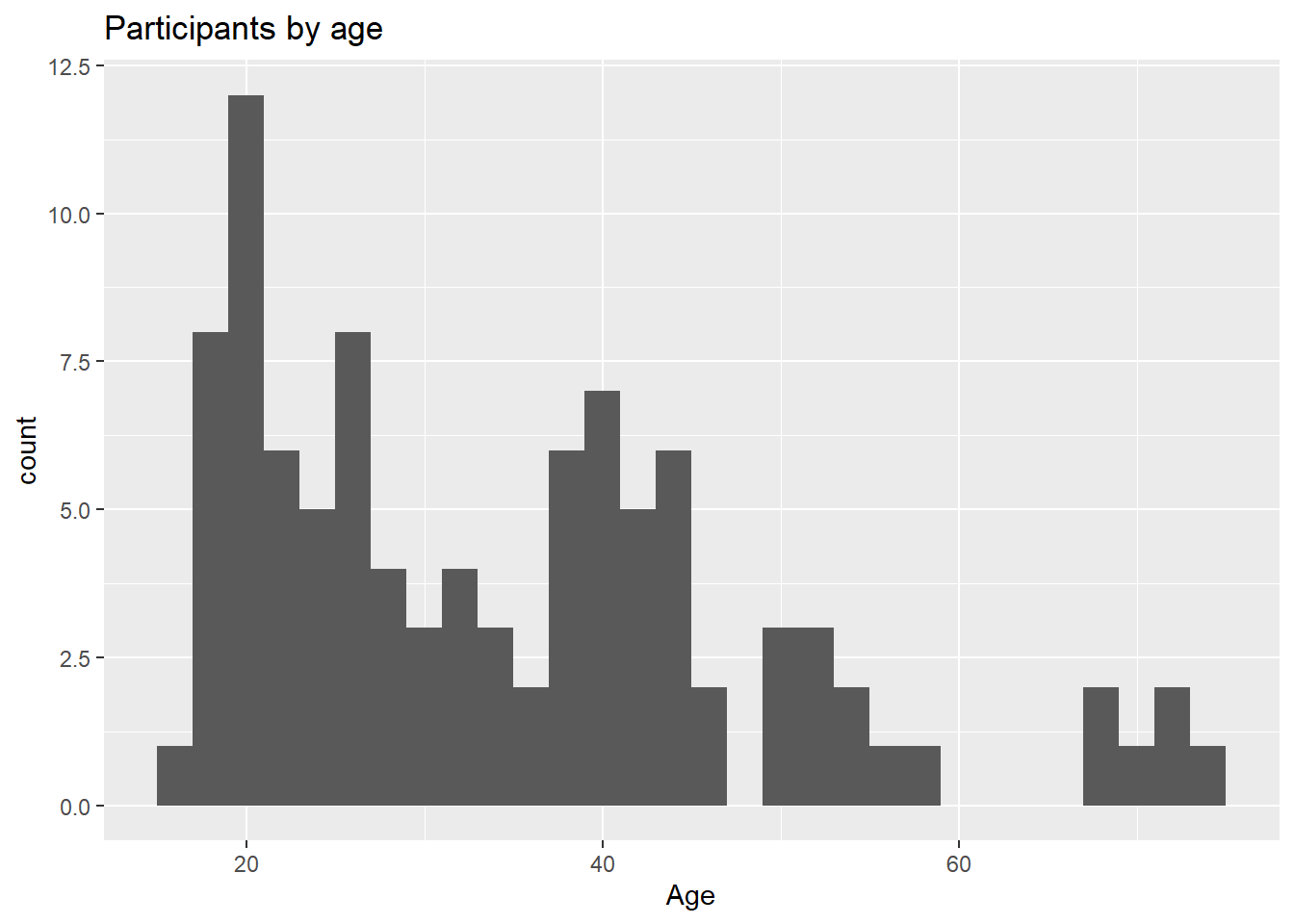Figure 3.15: Basic histogram

Most participants appear to be in their early 20’s with another group in their 40’s, and a much smaller group in their later sixties and early seventies. This would be a multimodal distribution.

Histogram colors can be modified using two options

• fill - fill color for the bars
• color - border color around the bars
# plot the histogram with blue bars and white borders
ggplot(Marriage, aes(x = age)) +
geom_histogram(fill = "cornflowerblue",
color = "white") +
labs(title="Participants by age",
x = "Age")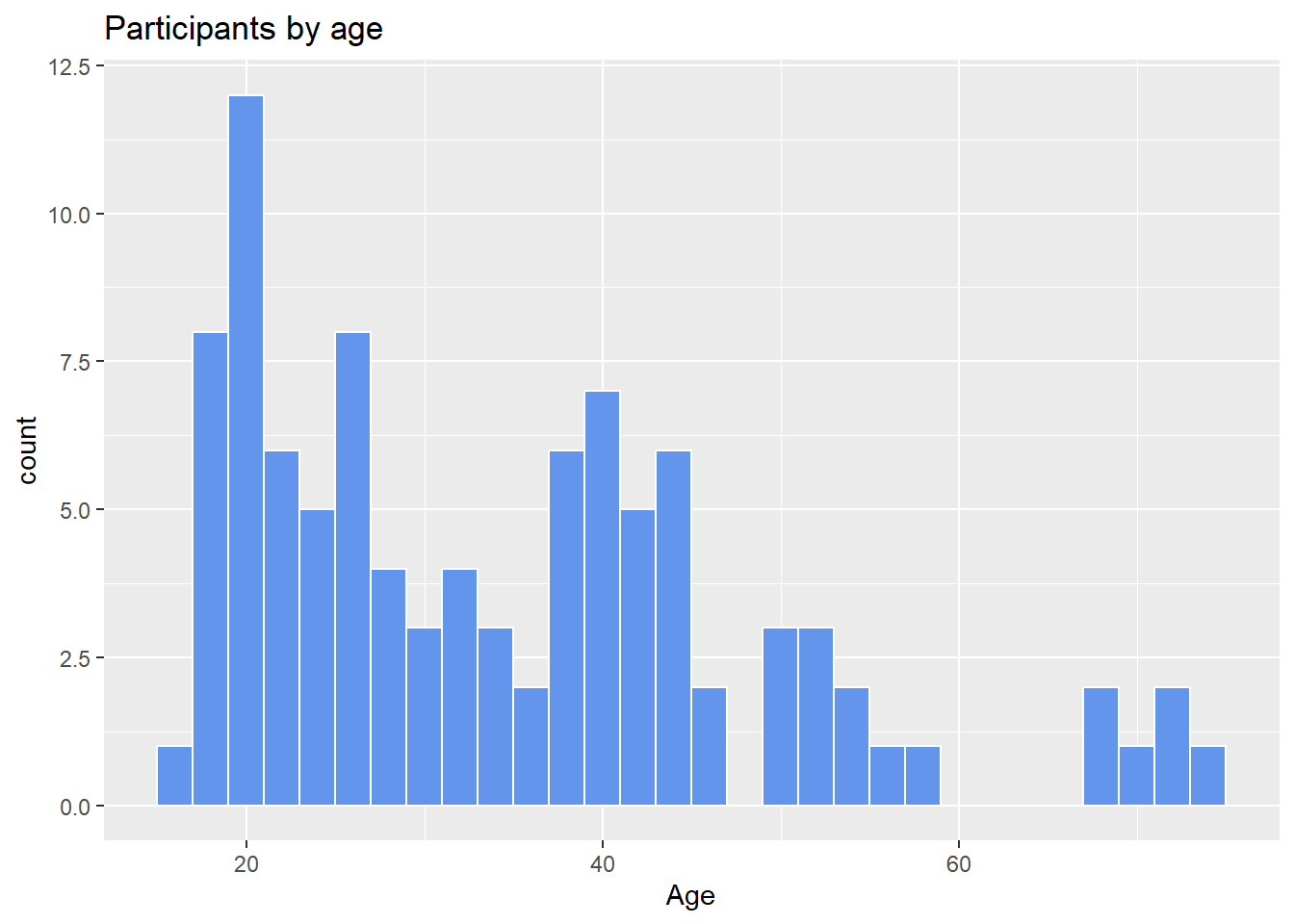Figure 3.16: Histogram with specified fill and border colors

#### 3.2.1.1 Bins and bandwidths

One of the most important histogram options is bins, which controls the number of bins into which the numeric variable is divided (i.e., the number of bars in the plot). The default is 30, but it is helpful to try smaller and larger numbers to get a better impression of the shape of the distribution.

# plot the histogram with 20 bins
ggplot(Marriage, aes(x = age)) +
geom_histogram(fill = "cornflowerblue",
color = "white",
bins = 20) +
labs(title="Participants by age",
subtitle = "number of bins = 20",
x = "Age")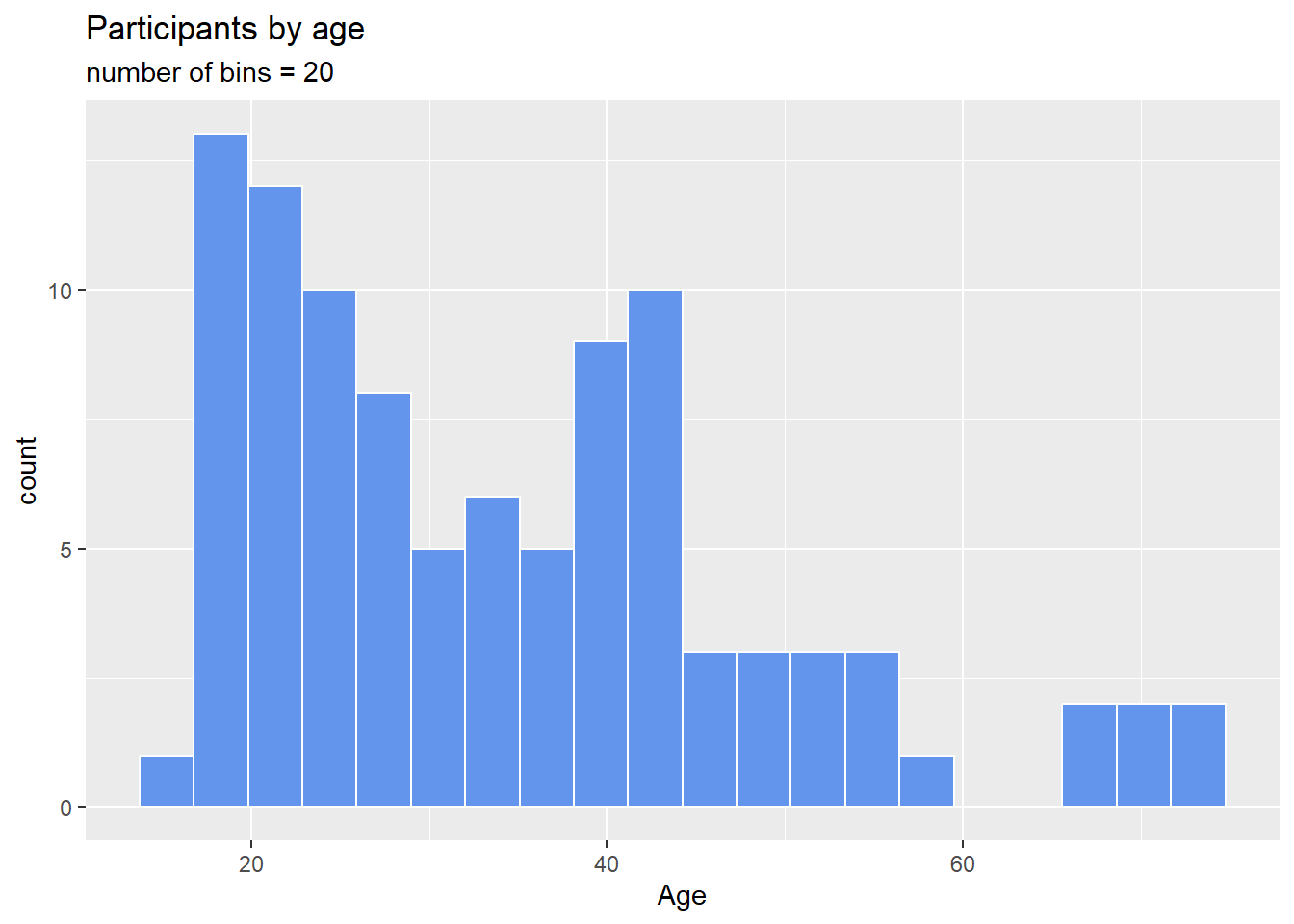Figure 3.17: Histogram with a specified number of bins

Alternatively, you can specify the binwidth, the width of the bins represented by the bars.

# plot the histogram with a binwidth of 5
ggplot(Marriage, aes(x = age)) +
geom_histogram(fill = "cornflowerblue",
color = "white",
binwidth = 5) +
labs(title="Participants by age",
subtitle = "binwidth = 5 years",
x = "Age")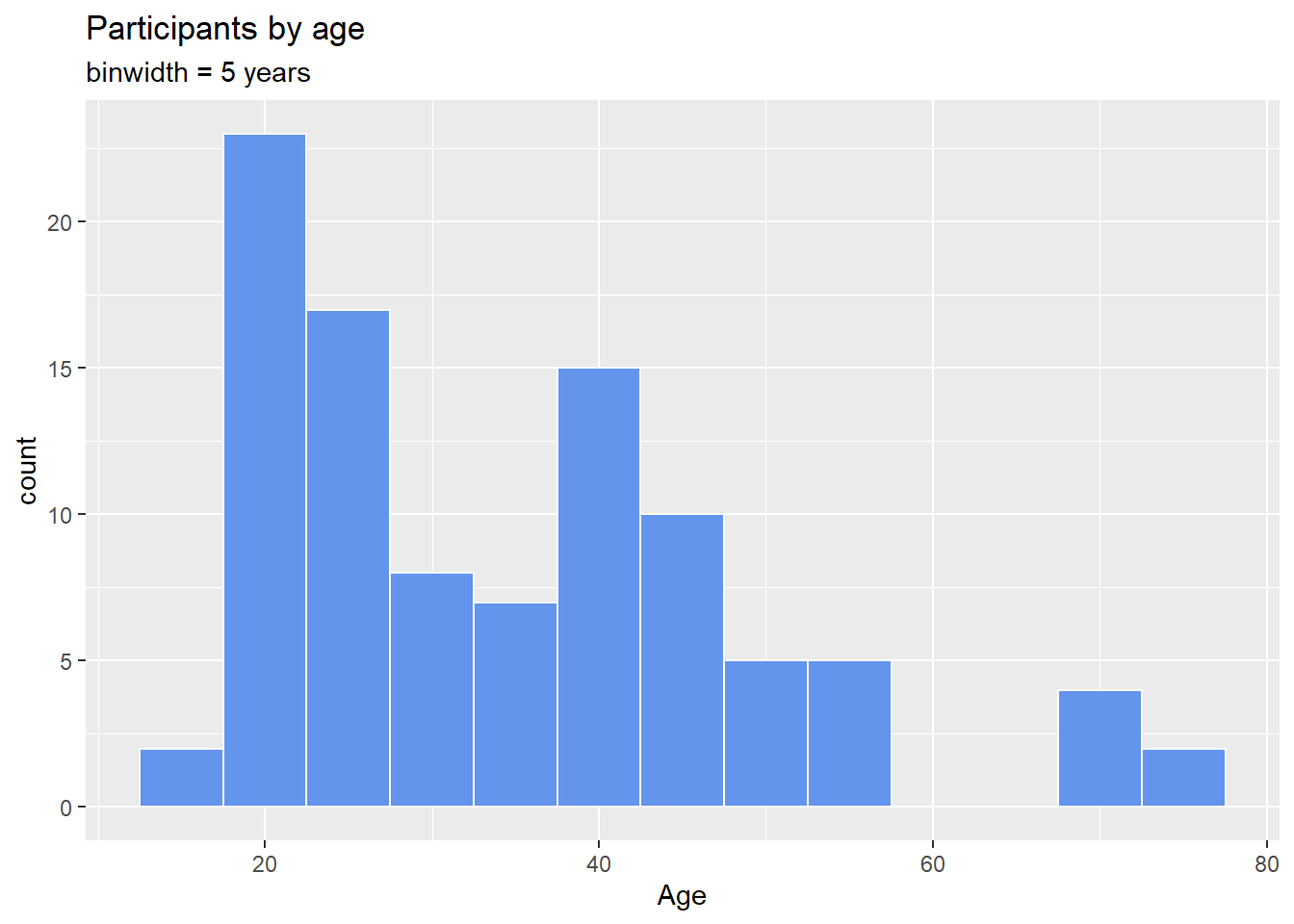Figure 3.18: Histogram with specified a bin width

As with bar charts, the y-axis can represent counts or percent of the total.

# plot the histogram with percentages on the y-axis
library(scales)
ggplot(Marriage,
aes(x = age,
y= ..count.. / sum(..count..))) +
geom_histogram(fill = "cornflowerblue",
color = "white",
binwidth = 5) +
labs(title="Participants by age",
y = "Percent",
x = "Age") +
scale_y_continuous(labels = percent)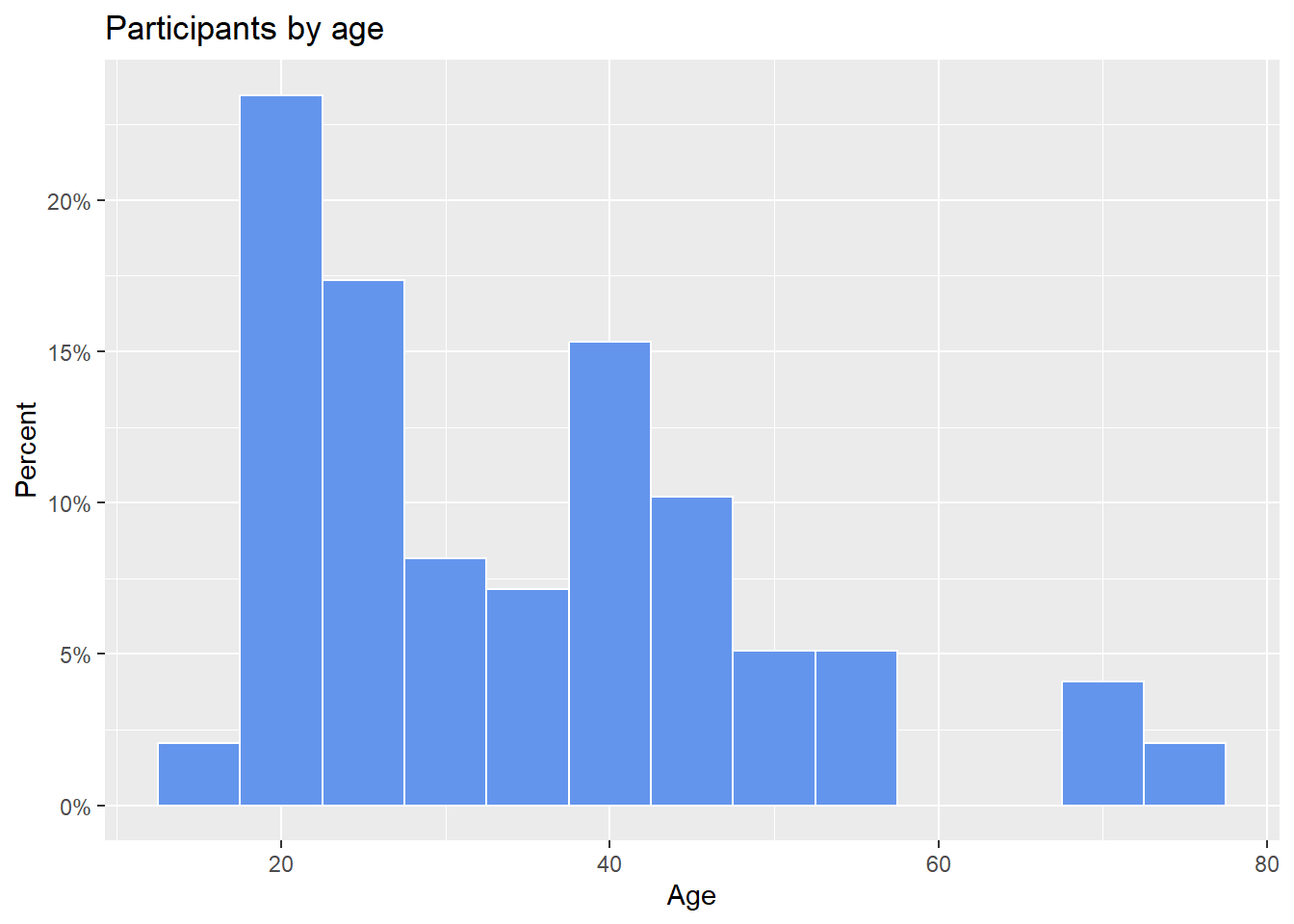Figure 3.19: Histogram with percentages on the y-axis

### 3.2.2 Kernel Density plot

An alternative to a histogram is the kernel density plot. Technically, kernel density estimation is a nonparametric method for estimating the probability density function of a continuous random variable. (What??) Basically, we are trying to draw a smoothed histogram, where the area under the curve equals one.

# Create a kernel density plot of age
ggplot(Marriage, aes(x = age)) +
geom_density() +
labs(title = "Participants by age")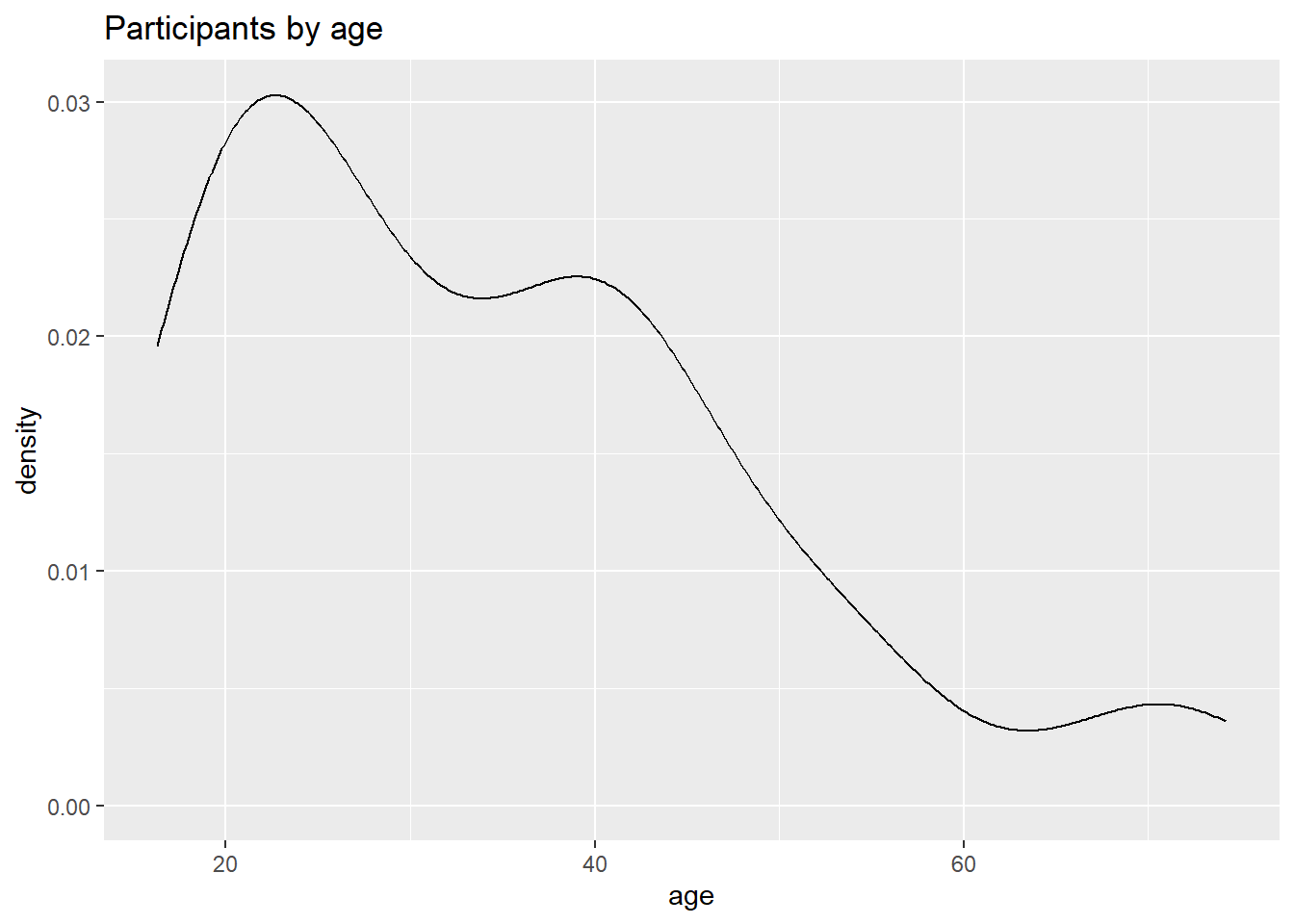Figure 3.20: Basic kernel density plot

The graph shows the distribution of scores. For example, the proportion of cases between 20 and 40 years old would be represented by the area under the curve between 20 and 40 on the x-axis.

As with previous charts, we can use fill and color to specify the fill and border colors.

# Create a kernel density plot of age
ggplot(Marriage, aes(x = age)) +
geom_density(fill = "indianred3") +
labs(title = "Participants by age")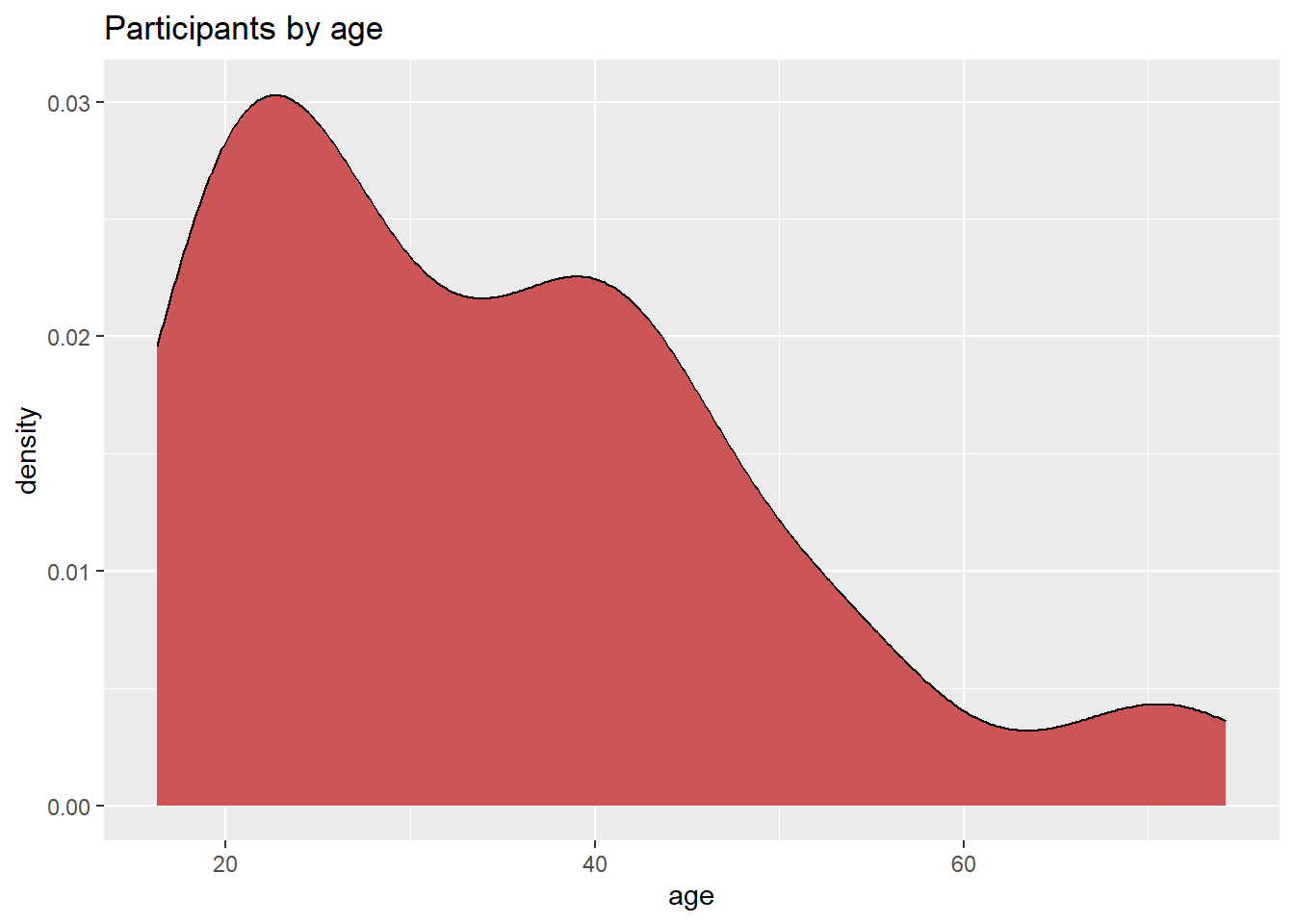Figure 3.21: Kernel density plot with fill

#### 3.2.2.1 Smoothing parameter

The degree of smoothness is controlled by the bandwidth parameter bw. To find the default value for a particular variable, use the bw.nrd0 function. Values that are larger will result in more smoothing, while values that are smaller will produce less smoothing.

# default bandwidth for the age variable
bw.nrd0(Marriage\$age)
##  5.181946
# Create a kernel density plot of age
ggplot(Marriage, aes(x = age)) +
geom_density(fill = "deepskyblue",
bw = 1) +
labs(title = "Participants by age",
subtitle = "bandwidth = 1")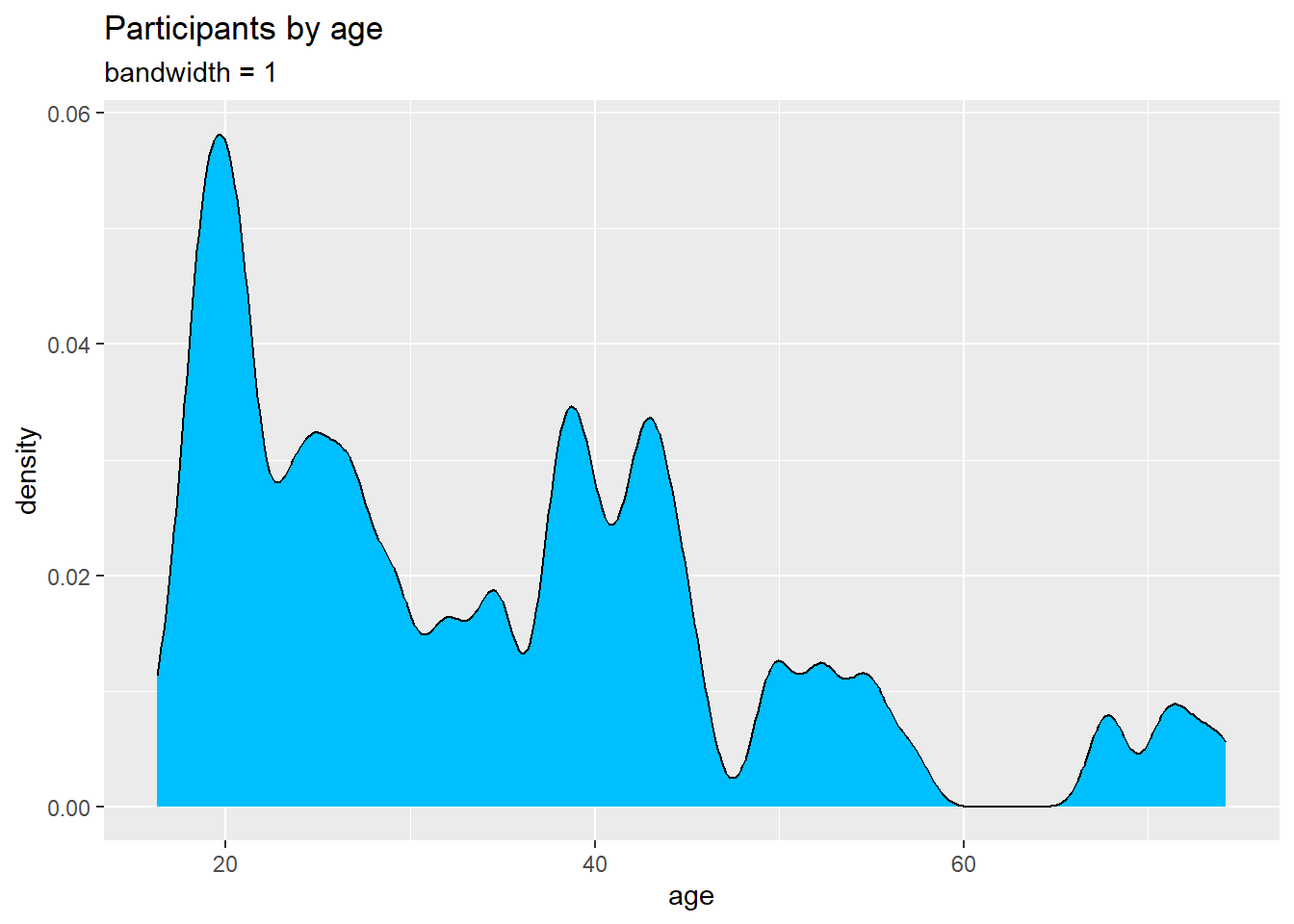Figure 3.22: Kernel density plot with a specified bandwidth

In this example, the default bandwidth for age is 5.18. Choosing a value of 1 resulted in less smoothing and more detail.

Kernel density plots allow you to easily see which scores are most frequent and which are relatively rare. However it can be difficult to explain the meaning of the y-axis to a non-statistician. (But it will make you look really smart at parties!)

### 3.2.3 Dot Chart

Another alternative to the histogram is the dot chart. Again, the quantitative variable is divided into bins, but rather than summary bars, each observation is represented by a dot. By default, the width of a dot corresponds to the bin width, and dots are stacked, with each dot representing one observation. This works best when the number of observations is small (say, less than 150).

# plot the age distribution using a dotplot
ggplot(Marriage, aes(x = age)) +
geom_dotplot() +
labs(title = "Participants by age",
y = "Proportion",
x = "Age")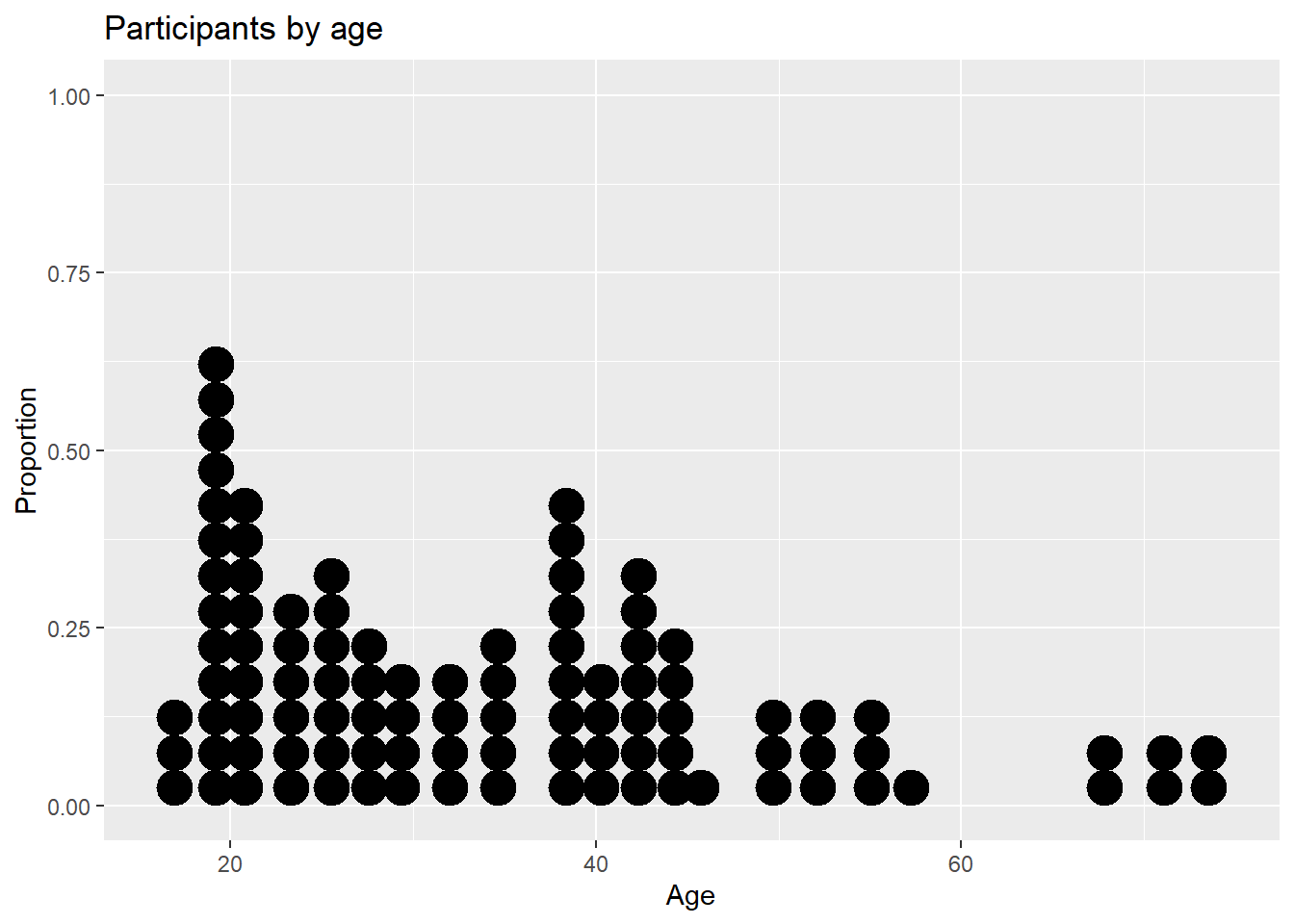Figure 3.23: Basic dotplot

The fill and color options can be used to specify the fill and border color of each dot respectively.

# Plot ages as a dot plot using
# gold dots with black borders
ggplot(Marriage, aes(x = age)) +
geom_dotplot(fill = "gold",
color = "black") +
labs(title = "Participants by age",
y = "Proportion",
x = "Age")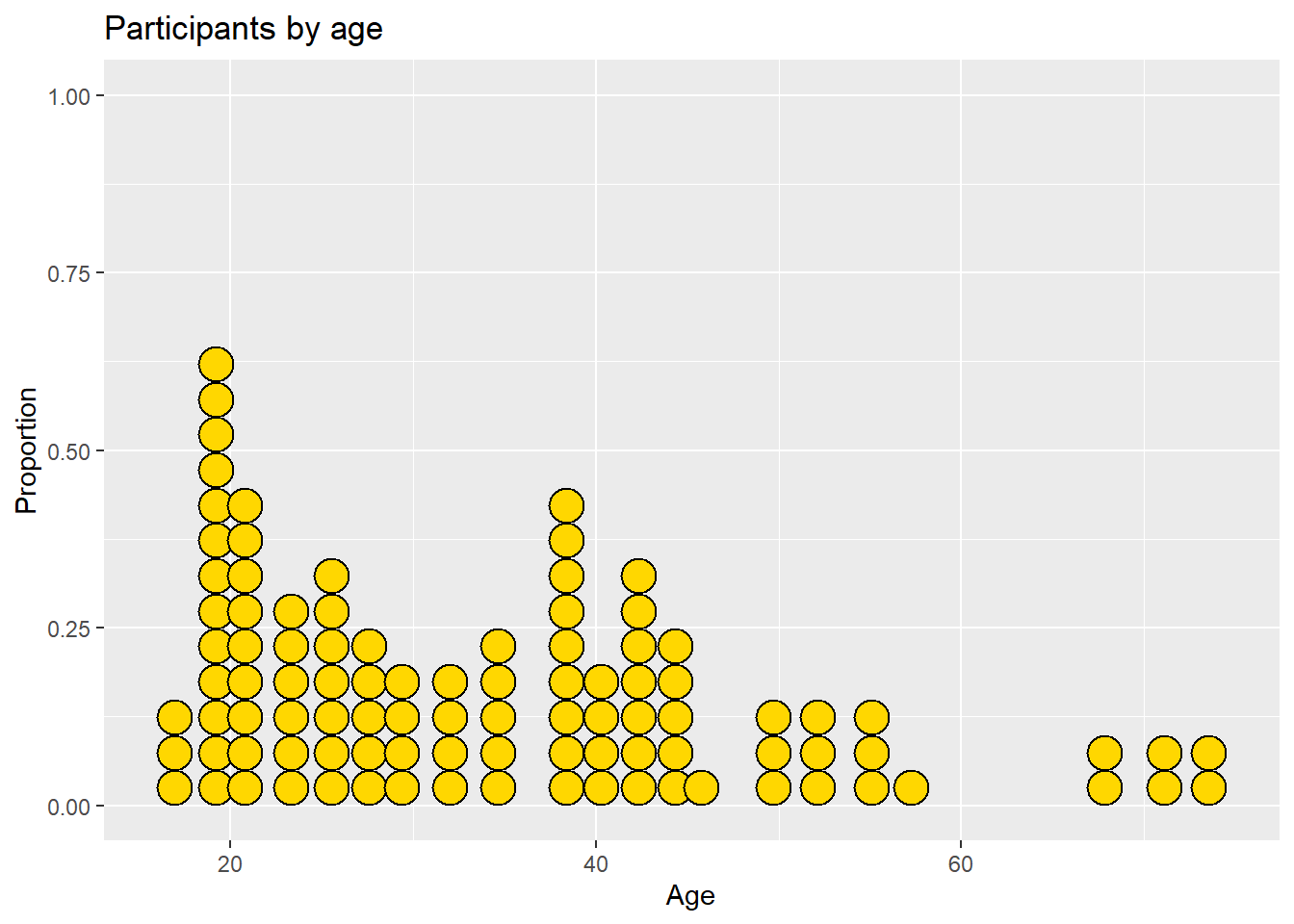Figure 3.24: Dotplot with a specified color scheme

There are many more options available. See the help for details and examples.